Electron. J. Diff. Equ., Vol. 2014 (2014), No. 86, pp. 1-12.

### Existence of solutions to nonlocal Kirchhoff equations of elliptic type via genus theory Nemat Nyamoradi, Nguyen Thanh Chung

Abstract:
In this article, we study the existence and multiplicity of solutions to the nonlocal Kirchhoff fractional equation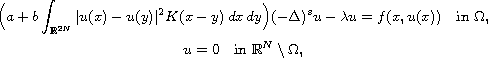where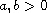are constants,is the fractional Laplace operator,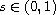is a fixed real number,is a real parameter and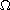is an open bounded subset of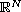,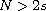, with Lipschitz boundary,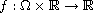is a continuous function. The proofs rely essentially on the genus properties in critical point theory.

Submitted December 15, 2013. Published April 2, 2014.
Math Subject Classifications: 34B27, 35J60, 35B05.
Key Words: Kirchhoff nonlocal operators; fractional differential equations; genus properties; critical point theory.

Show me the PDF file (267 KB), TEX file, and other files for this article.Nemat Nyamoradi Department of Mathematics, Faculty of Sciences Razi University, 67149 Kermanshah, Iran email: nyamoradi@razi.ac.ir, neamat80@yahoo.com Nguyen Thanh Chung Department of Mathematics, Quang Binh University 312 Ly Thuong Kiet, Dong Hoi, Quang Binh, Vietnam email: ntchung82@yahoo.com